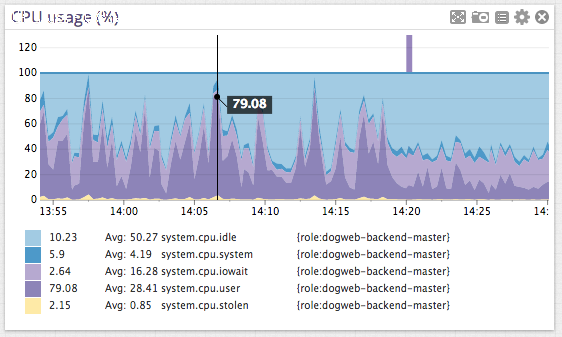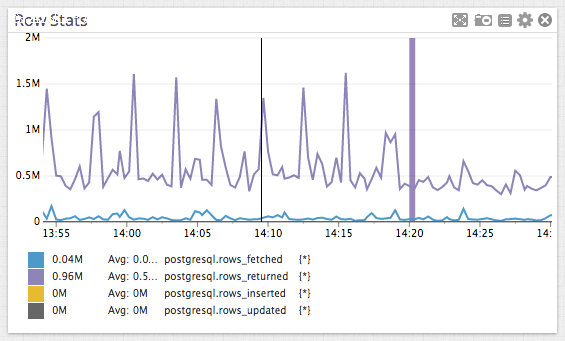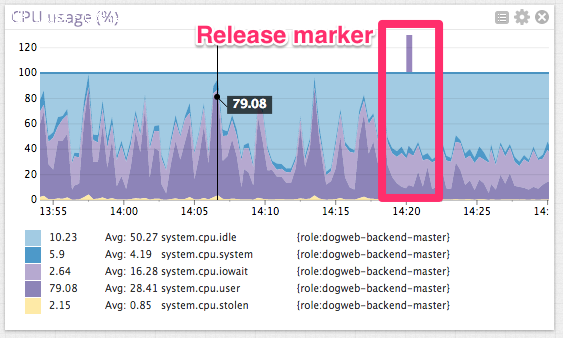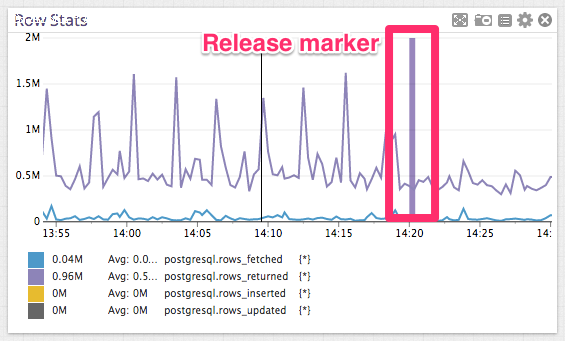Posted By：佳馨科技 Date：2014/3/15 13:25:18 最后修改：2014/3/15 13:25:18 Click Rate：6206

在PostgreSQL中修改了一行不明显的代码，把（ANY(ARRAY[...]) 改成 ANY(VALUES(...))），结果查询时间从20s变为0.2s。最初我们学习使用 EXPLAN ANALYZE来优化代码，到后来，Postgres社区也成为我们学习提升的一个好帮手，付出总会有回报，我们的性能也因此得到了极大的提升。

事出有因

查询与计划

结果令人震惊，罪魁祸首竟然是下面这个简单的查询：

```SELECT c.key,
c.x_key,
c.tags,
x.name
FROM context c
JOIN x
ON c.x_key = x.key
WHERE c.key = ANY (ARRAY[15368196, -- 11,000 other keys --)])
AND c.x_key = 1
AND c.tags @> ARRAY[E'blah'];```

X表拥有上千行数据，C表拥有1500万行数据，这两个表的“key”列都带有适当的索引主键。简单地说，它就是一个简单的主键查询。但有趣地是，随着key列中记录的增加，例如在11000行时，我们通过添加EXPLAIN (ANALYZE, BUFFERS)前缀来查看key列的值是否与数组中的值匹配：

```Nested Loop  (cost=6923.33..11770.59 rows=1 width=362) (actual time=17128.188..22109.283 rows=10858 loops=1)
Buffers: shared hit=83494
->  Bitmap Heap Scan on context c  (cost=6923.33..11762.31 rows=1 width=329) (actual time=17128.121..22031.783 rows=10858 loops=1)
Recheck Cond: ((tags @> '{blah}'::text[]) AND (x_key = 1))
Filter: (key = ANY ('{15368196,(a lot more keys here)}'::integer[]))
Buffers: shared hit=50919
->  BitmapAnd  (cost=6923.33..6923.33 rows=269 width=0) (actual time=132.910..132.910 rows=0 loops=1)
Buffers: shared hit=1342
->  Bitmap Index Scan on context_tags_idx  (cost=0.00..1149.61 rows=15891 width=0) (actual time=64.614..64.614 rows=264777 loops=1)
Index Cond: (tags @> '{blah}'::text[])
Buffers: shared hit=401
->  Bitmap Index Scan on context_x_id_source_type_id_idx  (cost=0.00..5773.47 rows=268667 width=0) (actual time=54.648..54.648 rows=267659 loops=1)
Index Cond: (x_id = 1)
Buffers: shared hit=941
->  Index Scan using x_pkey on x  (cost=0.00..8.27 rows=1 width=37) (actual time=0.003..0.004 rows=1 loops=10858)
Index Cond: (x.key = 1)
Buffers: shared hit=32575
Total runtime: 22117.417 ms```

这次查询共花费22s，我们可以通过下图对这22s进行很直观的了解，其中大部分时间花费在Postgres和OS之间，而磁盘I/O则花费非常少的时间。在最低水平，这些查询看起来就像是这些CPU利用率的峰值。在这里主要是想证实一个关键点：数据库不会等待磁盘去读取数据，而是做排序、散列和行比较这些事。

通过Postgres获取与峰值最接近的行数。显然，我们的查询在大多数情况下都有条不紊的执行着。

Postgres的性能问题：位图堆扫描

rows_fetched度量与下面的部分计划是一致的：

```Buffers: shared hit=83494
->  Bitmap Heap Scan on context c  (cost=6923.33..11762.31 rows=1 width=329) (actual time=17128.121..22031.783 rows=10858 loops=1)
Recheck Cond: ((tags @> '{blah}'::text[]) AND (x_key = 1))
Filter: (key = ANY ('{15368196,(a lot more keys here)}'::integer[]))
Buffers: shared hit=50919```

Postgres使用位图堆扫描（ Bitmap Heap Scan）来读取C表数据。当关键字的数量较少时，它可以在内存中非常高效地使用索引构建位图。如果位图太大，查询优化器会改变其查找数据的方式。在我们这个案例中，需要检查大量的关键字，所以它使用了非常相似的方法来检查候选行并且单独检查与x_key和tag相匹配的每一行。而所有的这些“在内存中加载”和“检查每一行”都需要花费大量的时间。

幸运的是，我们的表有30%都是装载在RAM中，所以在从磁盘上检查行的时候，它不会表现的太糟糕。但在性能上，它仍然存在非常明显的影响。查询过于简单，这是一个非常简单的key查找，所以没有显而易见的数据库或应用重构，它很难找到一些简单的方式来解决这个问题。最后，我们使用 PGSQL-Performance邮件向社区求助。

解决方案

开源帮了我们，经验丰富的且代码贡献量非常多的Tom Lane让我们试试这个：

```SELECT c.key,
c.x_key,
c.tags,
x.name
FROM context c
JOIN x
ON c.x_key = x.key
WHERE c.key = ANY (VALUES (15368196), -- 11,000 other keys --)
AND c.x_key = 1
AND c.tags @> ARRAY[E'blah'];```

你能发现有啥不同之处吗？把ARRAY换成了VALUES。

我们使用ARRAY[...]列举出所有的关键字来进行查询，但却欺骗了查询优化器。Values(...)让优化器充分使用关键字索引。仅仅是一行代码的改变，并且没有产生任何语义的改变。

下面是新查询语句的写法，差别就在于第三和第十四行。

```Nested Loop  (cost=168.22..2116.29 rows=148 width=362) (actual time=22.134..256.531 rows=10858 loops=1)
Buffers: shared hit=44967
->  Index Scan using x_pkey on x  (cost=0.00..8.27 rows=1 width=37) (actual time=0.071..0.073 rows=1 loops=1)
Index Cond: (id = 1)
Buffers: shared hit=4
->  Nested Loop  (cost=168.22..2106.54 rows=148 width=329) (actual time=22.060..242.406 rows=10858 loops=1)
Buffers: shared hit=44963
->  HashAggregate  (cost=168.22..170.22 rows=200 width=4) (actual time=21.529..32.820 rows=11215 loops=1)
->  Values Scan on "*VALUES*"  (cost=0.00..140.19 rows=11215 width=4) (actual time=0.005..9.527 rows=11215 loops=1)
->  Index Scan using context_pkey on context c  (cost=0.00..9.67 rows=1 width=329) (actual time=0.015..0.016 rows=1 loops=11215)
Index Cond: (c.key = "*VALUES*".column1)
Filter: ((c.tags @> '{blah}'::text[]) AND (c.x_id = 1))
Buffers: shared hit=44963
Total runtime: 263.639 ms```

从22000ms到200ms，仅仅修改了一行代码，速度提升了100倍还多。

产品里新的查询

部署后的代码：数据库看起来更美观Copyright © 2024  广西佳馨科技   地址：广西佳馨科技  电话：13878876479   QQ：120947546  电子邮件：1447876499@qq.com   站点提供API   桂ICP备14003626号-1